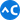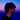# curry and compose in JavaScript

May 16, 2018

`curry` and `compose` are two of the very helpful functions that I started to use them at work on a daily basis.

## curry

In most functional languages, functions are curried by default. So you can use partial application out of the box. Here’s a piece of Haskell:

``````add :: Int -> Int -> Int
add x y = x + y

main = print (increment 41) -- 42``````

As described by its Hindley–Milner type signatures, `add` takes an integer (`x`) and returns a function `Int -> Int`. By passing another integer (that is `y`) to this function, it gives us the sum of the numbers.

Although in JavaScript functions are not curried by default, we can implement a helper to do that for us. `curry`:

``````function curry(fn) {
const arity = fn.length;

return function \$curry(...args) {
if (args.length < arity) {
return \$curry.bind(null, ...args);
}

return fn.call(null, ...args);
};
}``````

We can get the number of arguments that a function takes a.k.a. arity by getting the `length` of that function. We keep checking to see if we have the number of arguments we need to execute the original function `fn`, if we didn’t, we return a function with the given arguments already applied.

Here’s the JavaScript version of `add`

``````add = curry((a, b) => a + b);

increment(41) // 42``````

## compose

The idea behind `compose` is, well, to compose functions. You build small reusable functions and compose them together to solve a more complex problem.

Here’s a piece of Haskell that composes two functions.

``````isOdd = not.even

main = print (isOdd 9) -- True``````

We pass the result of `even` to `not`.

JavaScript doesn’t have anything built-in to help us with that. Although there’s a proposal for a pipe operator that can help us to get a similar functionality, for now we can build something with what we already have. `compose`:

``````function compose(...fns) {
const n = fns.length;

return function \$compose(...args) {
let \$args = args;

for (let i = n - 1; i >= 0; i -= 1) {
\$args = [fns[i].call(null, ...\$args)];
}

return \$args;
};
}``````

We run a loop and accumulate the result of calling all the provided functions, from right to left. The returned value of each function is the argument of the next function (again from right to left).

Let’s write `isOdd` in JavaScript

``````const even = x => x % 2 === 0;
const not = x => !x;
const isOdd = compose(not, even);

isOdd(9) // true``````

if the passed functions are curried, we can do even more beautiful stuff:

``````// helper methods from ramdajs

const hexToDecimal = flip(curry(parseInt))(16);

const getColor = compose(
map(hexToDecimal),
splitEvery(2),
replace("#", ""),
prop("color")
);

getColor({ color: "#1d97ff" }); // [29, 151, 255]``````

## Conclusion

`curry` and `compose` help us to think in a more modular way. They improve the readability and reusability of code. If you want to go deeper, I recommend reading currying and Coding by Composing. If you have anything to say I’m fhdhsni on twitter.